# Pedestrian up-down hill

Pedestrian goes for a walk first at plane at 4 km/h, then uphill 3 km/h. Then it is in the middle of the route, turns back and goes downhill at speed 6 km/h. Total walk was 6 hours. How many kilometers went pedestrian?

Result

s =  24 km

#### Solution:

6 = a+b+c+d
s = 4a+3b+6c+4d
4a=4d
3b=6c
a=0

a+b+c+d = 6
4a+3b+6c+4d-s = 0
4a-4d = 0
3b-6c = 0
a = 0

a = 0
b = 4
c = 2
d = 0
s = 24

Calculated by our linear equations calculator.

Leave us a comment of example and its solution (i.e. if it is still somewhat unclear...):Be the first to comment!#### To solve this verbal math problem are needed these knowledge from mathematics:

Looking for help with calculating harmonic mean? Looking for a statistical calculator? Do you have a linear equation or system of equations and looking for its solution? Or do you have quadratic equation? Do you want to convert length units?

## Next similar examples:

1. Cyclist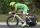A cyclist is moving at 35 km/h and follow pedestrian who is walking at speed 4 km/h. Walker has an advantage 19 km. How long take cyclist to catch up with him?
2. Cyclist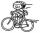Cyclist goes uphill 10 km for 50 minutes and downhill minutes for 29 minutes, both applied to the pedals same force. How long he pass 10 km by plane?
3. Dog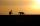Man goes with a dog on a long walk 17 km from the house. Man is walking at speed 4.9 km/h and a dog that constantly running between house and man at 12.6 km/h. How many kilometers will run the dog when they reach their destination?
4. Forth and back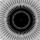Car goes from point A to point B at speed 78 km/h and back at 82 km/h. If went there and back at speed 81 km/h journey would take five minutes less. What is the distance between points A and B?
5. Scouts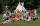The boys from scout group traveled 5 days distance 115 km. Every day walked 1.5 km less than the previous day. How many kilometers scouts walked in the first day?
6. Two carsTwo cars started against each other at the same time to journey long 293 km. First car went 41 km/h and second 41 km/h. What distance will be between this cars 20 minutes before meet?
7. The cyclist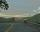The cyclist went from village to town. First half of journey went at 20 km/h. The second half of the journey, which mostly fell, went at 39 km/h. All journey took 88 minutes. Calculate the distance from the village to the town.
8. Tourists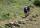From the cottage started first group of tourists at 10:00 AM at speed 4 km/h. The second started after them 47 minutes later at speed 6 km/h. How long and how many kilometers from the cottage will catch the first group?
9. Two runners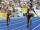Two runners ran simultaneously towards each other from locations distant 34.6 km. The average speed of the first runner was 1/5 higher than the average speed of the second runner. How long should each ran a 34.6 km, if you know that they meet after 67 mi
10. Train and car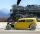The train and the car started at a constant speed to journey. When the train travels 87 km, the car travels 97 km. How many km travels the train when the car travels 87 km?
11. Rotation of the EarthCalculate the circumferential speed of the Earth's surface at a latitude of 61°​​. Consider a globe with a radius of 6378 km.
12. Speed of Slovakian trainsRudolf decided to take the train from the station 'Trnava' to 'Zemianske Kostoľany'. In the train timetables found train R 725 Remata : km0Bratislava hl.st.12:574Bratislava-Vinohrady13:0113:0219Pezinok13:1213:1346Trnava13:3013:3263Leopoldov13:4514:0168H
13. Map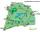On the tourist map at a scale of 1 : 50000 is distance between two points along a straight road 3.7 cm. How long travels this distance on a bike at 30 km/h? Time express in minutes.
14. TrainsFrom station 130 km away started passenger train and after 2.2 hours after the express train, which travels 37 km an hour more. Express train finish journey 7 minutes early. Calculate the average speed of this two trains.
15. Free fall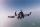Lloyd fall from height 7 m. Calculate the speed he hit the ground when falling with acceleration g = 9.81 m/s2
16. Train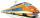The train is running at speeds of 96 km/h. From the beginning of braking to full stop train run for 3.3 minutes. If the train slows the braking equally, calculate the distance of the place from the station where you need to start to brake.
17. Average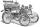What is the average speed of the car, where half of the distance covered passed at speed 66 km/h and the other half at 86 km/h.